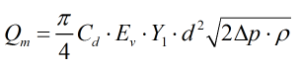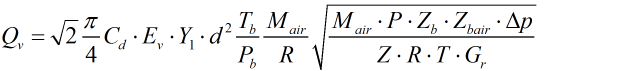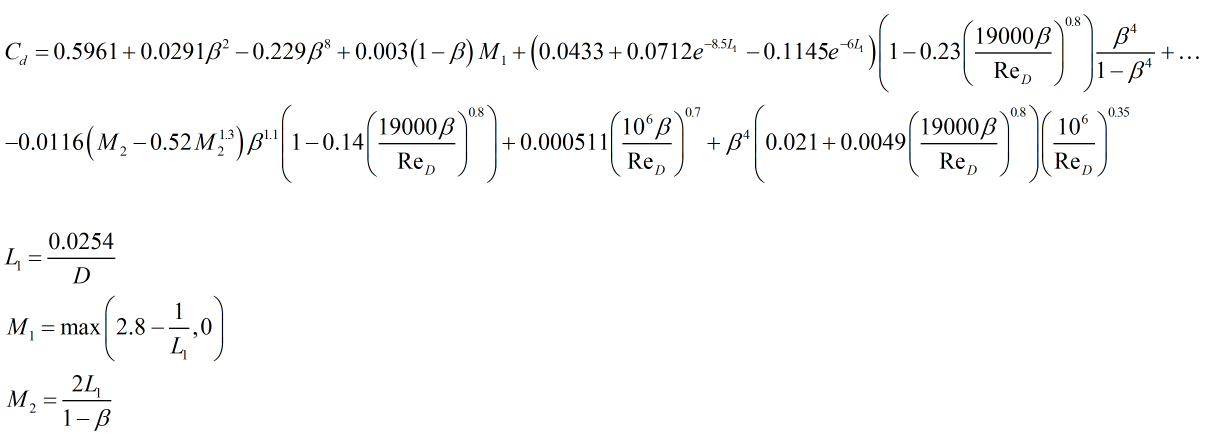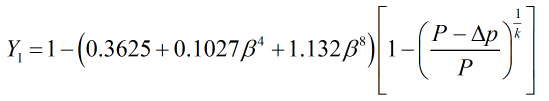# C285 – AGA 3:2012 – Orifice Plate Flow Calculation

## Description

This calculates the flow rate measured through an orifice plate metering system. The calculation can also be rearranged to solve the differential pressure across an orifice plate or the bore diameter.

AGA Report No.3 – Orifice Metering of Natural Gas and Other Related Hydrocarbon Fluids – Concentric, Square-Edged Orifice Meters – Part 1: General Equations and Uncertainty Guidelines (2012)

Kelton calculation reference C285

FLOCALC calculation reference F098

KIMS Calculation reference K232

## Options

### Fluid

• Gas
• Liquid

This option is used to select whether the fluid is liquid or a gas. If it is liquid the expansibility factor will be set to 1.

### Solve for

• Flow
• Orifice size
• Differential pressure

This option is used to select the desired output of the calculation.

### Pressure

• Measured at upstream tap (P1)
• Measured at downstream tap (P2)

This option is available when solving for flow rate. It allows the user the option of inputting pressure at either the upstream or downstream tap.

### Solution method

• Direct substitution
• Newton-Raphson

This option is used to select the method of calculation used to gain the flow rate. Both methods are generally acceptable to use though the Newton-Raphson converges faster. The direct substitution method should not be used for high viscosity liquids.

### Method

• Developed from the density of fluid
• Developed from the real gas relative density

This option allows the user to select which set of inputs they wish to use for implementation of the AGA 3 flow rate equations. Both sets have common inputs such as geometry of the system and differential pressure, dynamic viscosity etc, but the first requires line density, the second uses real gas relative density along with PTZ data.

The remaining options will be drawn are seen in C261 or C262 and depend on the fluid used.

### Calculate for

• Mass
• Observed volume
• Standard volume
• Normal volume
• Energy

### Calculate from

• Mass
• Observed volume
• Standard volume
• Normal volume

This option becomes active if solving for an orifice size or differential pressure

### Constants

Molecular mass of air – Mair = 28.9625 g/mol

Gas constant – R = 8.314472 J/molK

## Calculation

### Mass flow rate

Mass flow rate as calculated ‘from the density of the fluid’ is derived from equation 3-1 in AGA 3 Part 3.Where Cd = Coefficient of discharge β = Diameter ratio ε = Expansibility factor d = Orifice diameter Δp = Differential pressure ρ = Line density

### Standard volume flow rate

Standard volume flow rate as calculated ‘from the real gas relative density’ is done derived from equation 3-6a in AGA 3 Part 3.Where Cd = Coefficient of discharge Ev = Velocity of approach factor ε = Expansibility factor d = Orifice diameter Tb = Base temperature Pb = Base pressure Mair = Molecular weight of air R = Gas Constant P = Line pressure Z = Line compressibility Zb = Base compressibility Zbair = Base compressibility of air T = Line temperature Gr = Real gas relative density Δp = Differential pressure

### Coefficient of Discharge

AGA 3 uses the Reader-Harris Gallagher equation for the calculation of coefficient of discharge, Cd, this is derived from equations 3-11 through to 3-21 in AGA 3 Part 3 and is as follows.Where β = Diameter ratio ReD = Reynolds number D = Pipe diameter in metres

### Expansion Factor

The expansion factor used in the 2012 revision of the AGA 3 standard is as follows:Where P = Line pressure Δp = Differential pressure β = Diameter ratio k = Isentropic exponent

### Velocity of Approach Factor

The velocity of approach factor is calculated by:Where β = Diameter ratio

Back